Next: Linear constraints Up: Constrained Optimization Previous: Constrained Optimization   Contents

# A short review of the available techniques.

In the industry, the objective function is very often a simulator of a complex process. The constraints usually represents bounds on the validity of the simulator. Sometimes the simulator can simply crash when evaluating an infeasible point (a point which do not respects the constraints). For this reason, the optimization algorithm generates only feasible points (due to rounding errors, some points may be infeasible especially when there are non-linear constraints. Anyway, the generated infeasible points are always very close to feasibility ).
There are two possible approaches. The first approach is now described.
The steps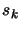of the unconstrained algorithm are the solution of: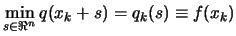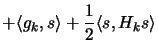(8.1) subject to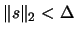In the first approach (=approach 1"), the stepof the constrained algorithm are the solution of:(8.2)

The problem of solving 8.2 is not trivial at all. It's in fact as difficult as the original problem. The only advantage in solving this subproblem at each step is that the objective function (which is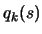) evaluations are cheap and thus we can have very precise steps leading (hopefully) to a fast convergence to the solution of the original problem.
The same methods used for solving the subproblem 8.2 can be directly applied to the original non-linear objective function. This is our second approach (=approach 2").
There are specific methods for box or linear constraints and for non-linear constraints. We will describe them in two separate chapters.

SubsectionsNext: Linear constraints Up: Constrained Optimization Previous: Constrained Optimization   Contents
Frank Vanden Berghen 2004-04-19